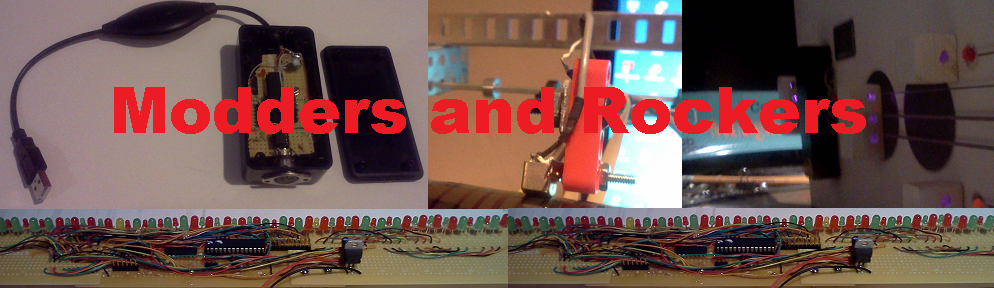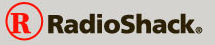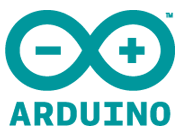# LittleBits 'Softbits' for the Arduino

power_on(int chain)
Starts a chain of bits.
power_off()
Optional end of a chain module.

input_d0()
Read the d0 digital input. Output is 0 or 255.
input_a0()
input_a1()

output_d1()
Output to d1 digital output. Output is 0v or +5v.
output_a5()
Output to d5 as analog output. Output is any value from 0v to +5v.
Switch in analog position.
output_a9()
Output to d9 as analog output. Output is any value from 0v to +5v.
Switch in analog position.
output_d5()
Output to d5 as digital output. Output is 0v or +5v.
output_d9()
Output to d9 as digital output. Output is 0v or +5v.

arith_plus(int chain)
Add current value to the value from the specified chain. Output is limited to any value from 0 to 255.
arith_minus(int chain)
Subtract value from specified chain from the current value. Output is limited to any value from 0 to 255.
arith_times(int chain)
Multiply current value by the value from the specified chain. Output is limited to any value from 0 to 255.
arith_divide(int chain)
Divide the current value by the value from the specified chain. Output is limited to any value from 0 to 255.

logic_and()
Output value is 255 if both the current value AND the value from the specified chain are greater than 127 else it is 0. Values greater than 127 are treated as TRUE and values less than or equal to 127 is treated as FALSE.
logic_or()
Output value is 255 if either the current value OR the value from the specified chain are greater than 127 else it is 0. Values greater than 127 are treated as TRUE and values less than or equal to 127 is treated as FALSE.
logic_not()
Output value is 255 if the current value is less than or equal to 127 else the output is 255. Values greater than 127 are treated as TRUE and values less than or equal to 127 is treated as FALSE. This has the same functionality as the LittleBits w10 inverter bit
logic_nand()
Output value is 0 if both the current value AND the value from the specified chain are greater than 127 else it is 255. Values greater than 127 are treated as TRUE and values less than or equal to 127 is treated as FALSE. The output has the same value as a logic_and() followed by a logic_not().
logic_nor()
Output value is 0 if either the current value OR the value from the specified chain are greater than 127 else it is 255. Values greater than 127 are treated as TRUE and values less than or equal to 127 is treated as FALSE. The output has the same value as a logic_or() followed by a logic_not().

wire_split(int chain)
Copy the current value to the start value of the specified chain.
wire_loop()
Copy the current value to the start value of the current chain.

power_on(int chain)
The purpose of the power on function is to initialize a new chain of modules. It copies the start value for the specified chain to the current value. It sets the chain number and the current chain number.
power_off()
The purpose of the power off function is to do any cleanup at the end of a chain. It sets the current chain number to 0 and the chain number to 0. Calls to Softbits modules before a power on or after a power off will do nothing since they check the value of current chain and if it is zero they exit.
input_d0()
Read the d0 digital input. If it is HIGH then set the current value to 255. If it is LOW then set the current value to 0.
input_a0()
Read the a0 analog input. The analog input returns a 10 bit value that goes from 0 to 1023 as the input voltage goes from 0 volts to 5 volts. The value is divided by four to give an 8 bit value that goes from 0 to 255.
input_a1()
Read the a1 analog input. The analog input returns a 10 bit value that goes from 0 to 1023 as the input voltage goes from 0 volts to 5 volts. The value is divided by four to give an 8 bit value that goes from 0 to 255.
output_d1()
Output to d1 digital output. If the current value is greater than 127 then the output is set HIGH. If the current value is less than or equal to 127 then the output is set LOW. The use_d1() function needs to be specified in the setup() function.
output_a5()
Output to d5 as analog output. Output is any value from 0v to +5v.
Switch in analog position.
output_a9()
Output to d9 as analog output. Output is any value from 0v to +5v.
Switch in analog position.
output_d5()
Output to d5 as digital output. Output is 0v or +5v.
output_d9()
Output to d9 as digital output. Output is 0v or +5v.
arith_plus(int chain)
Add current value to the value from the specified chain. Output is limited to any value from 0 to 255.
arith_minus(int chain)
Subtract value from specified chain from the current value. Output is limited to any value from 0 to 255.
arith_times(int chain)
Multiply current value by the value from the specified chain. Output is limited to any value from 0 to 255.
arith_divide(int chain)
Divide the current value by the value from the specified chain. Output is limited to any value from 0 to 255.
wire_split(int chain)
Copy the current value to the start value of the specified chain.
wire_loop()
Copy the current value to the start value of the current chain.
logic_and()
Output value is 255 if both the current value AND the value from the specified chain are greater than 127 else it is 0. Values greater than 127 are treated as TRUE and values less than or equal to 127 is treated as FALSE.
logic_or()
Output value is 255 if either the current value OR the value from the specified chain are greater than 127 else it is 0. Values greater than 127 are treated as TRUE and values less than or equal to 127 is treated as FALSE.
logic_not()
Output value is 255 if the current value is less than or equal to 127 else the output is 255. Values greater than 127 are treated as TRUE and values less than or equal to 127 is treated as FALSE. This has the same functionality as the LittleBits w10 inverter bit
logic_nand()
Output value is 0 if both the current value AND the value from the specified chain are greater than 127 else it is 255. Values greater than 127 are treated as TRUE and values less than or equal to 127 is treated as FALSE. The output has the same value as a logic_and() followed by a logic_not().
logic_nor()
Output value is 0 if either the current value OR the value from the specified chain are greater than 127 else it is 255. Values greater than 127 are treated as TRUE and values less than or equal to 127 is treated as FALSE. The output has the same value as a logic_or() followed by a logic_not().